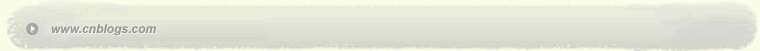Impossible is nothing 爱过知情重醉过知酒浓 　　花开花谢终是空 　　缘份不停留像春风来又走 　　女人如花花似梦

 < 2022年10月 >
2526272829301
2345678
9101112131415
16171819202122
23242526272829
303112345

• 随笔 - 8
• 文章 - 91
• 评论 - 16
• 引用 - 0

# 相册

•

### 评论排行榜#include<iostream>#include
<string>using namespace std;void main(){char *a="010000010111101101000000000";int i,temp0=0,temp1=0,max0=0,max1=0;//对变量初始化，如果不初始化，变量值是随机的for(i=0;i<strlen(a);i++){switch(a[i]){case '0':{temp0
++;if(a[i+1]=='1'||i==strlen(a)-1){if(max0<temp0) max0=temp0;temp0
=0;}break;}case '1':{temp1
++;if(a[i+1]=='0'||i==strlen(a)-1){if(max1<temp1) max1=temp1;temp1
=0;}break;}}}cout
<<"max0="<<max0<<endl;cout
<<"max1="<<max1<<endl;}

#include <iostream>
void main()
{
char *a="010000010111101101000000000";
int max = {0, 0};
int count = {0, 0};

while(*a)
{
int index = *a - '0';
count[index] ++;
count[index^1] = 0; // count[index?0:1] = 0; // count[index==0?1:0] = 0;
if(count[index] > max[index])
max[index] = count[index];
a++;
}
std::cout << "max 0: " << max << std::endl;
std::cout << "max 1: " << max << std::endl;
}

// output
// max 0: 9
// max 1: 4

1. 就C字串来说，从头到尾的遍历不需要for，用while是最佳选择。
2. strlen是很浪费的操作，如果非要用，对同一个不变长度的字串来说，用一个变量来存储，然后重复使用，比重复计算strlen要好得多。
3. 对数字串来说，可以直接将字符减去'0'得到相应的数字。
4. 比较判断其实并不比赋值省时，有时候直接赋值，比起比较后再赋值可能更有效率。更何况你的判断条件达三条之多才决定是否需要赋值。

5. 利用数组的下标，会给你带来意想不到的简洁。

6. 最后，变量没有初始化，是算法设计的问题。如果初始条件都没有确定，只能说明你的算法没有想清楚。
posted on 2006-03-02 22:37 笑笑生 阅读(152) 评论(0)  编辑 收藏 引用 所属分类: C++语言只有注册用户登录后才能发表评论。 【推荐】超50万行VC++源码: 大型组态工控、电力仿真CAD与GIS源码库 相关文章:Copyright © 笑笑生 Powered by: 博客园 模板提供：沪江博客